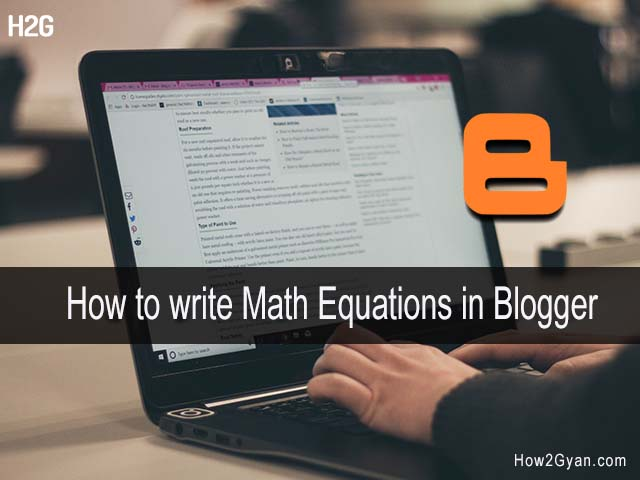# How to Add Equations in Blogger BlogHow to add Equations in Blogger Blog? Today in this blog post you will learn how to write Math Equations in a Blog post (Blogger) with a simple step-by-step including lots of screenshot instruction guide.

If you are a teacher and like to teach Mathematics online with a Blog host on Blogger. It's free and easy to create a teaching Blog in Blogger.

But one thing that will disappoint you is that there is no plugin available to insert a Mathematics equation in Blogger.

You know that for a Math tutorial Blog Mathematics equations are the most important and without equations Math Blog is meaningless.

So through this blog post, I want to share some useful methods that probably help you a lot to insert a Mathematical equation easily in your blog post.

## How to Add Equations in Blogger (Blogspot)

Here in this post, I have shared two methods through which you can insert or add Mathematical equations or any Chemical equations on your Blogger blog. Inserting an equation into your Blogger (Blogspot) blog is easy, just follow this post's steps till the end.

Let's start...

### Method 1: Insert Equations as Image

The easiest way to insert a Mathematics equation into the Blogger post that I found is to Insert the Mathematics equations as an image. For that first, you have to write the equation in the PowerPoint slide and save it in an image format (PNG or JPEG).

After that come to Blogger post and click the Image icon then upload the image. Follow the steps to insert the Math equation into a PowerPoint slide and save it as an image format.

To insert a Mathematical equation in a Blogger post as an image follow the steps below:

Step 1: Open any version of PowerPoint with the Blank template (presentation)
Change the slide layout to Blank [ Home > Right-click on slide > Layout > Blank ]

Step 2: Now click on the Insert tab then click on the Equation option and type the equation using Structures and Symbols

Step 3: After your equation is ready, go to the File tab > Save As Computer > Browse (select a file location to save the file) > PNG (or JPEG) format > Save

Now you have to just upload that image to the Blogger (Blogspot) server. Read,  Once you upload the equation will be seen in your blog post as an image.

You may also like to read how to insert a table in Blogger

### Method 2: Using Online Math Equation editors

You can also insert equations to Blogger using an online Mathematics equation editor. There are so many online Math equation editor websites available that provide you free services to create a Mathematics equation online.

Here below how you can insert the Math equation using the online Math Equation Editor CodeCogs.Com, follow the steps:

Step 1: Go to the link Latex.codecogs and click the fx icon

Step 2: Then there will open a CodeCogs Equation Editor. Now type the equation using these tools. After all, do hit the Ok button

Step 3: Now you will see that equation on the example box. Select the equation and press Ctrl + C and paste (Ctrl + V) in the post section where you want to show the equation.

That's done the equation you want to insert in your post is now successfully added.

Here is a list of free online Mathematics equation editor websites like CodeCogs
• Latex4technics.com
• Math.typeit.org
• Sciweavers.org
• atomurl.net
• mathcha.io

That's all about how you can easily insert a Mathematical equation in the Blogger post.

Using one of these three methods mentioned in this article you can easily insert any kind of Math and Chemical reaction equations on your Blogger post such that it makes your blog most helpful to readers.

I hope this post should help you to insert Equations in your Blogger Blogspot blog.

If you like to read our blog posts then, please share them on social platforms - Facebook, Twitter, WhatsApp, etc. And also feel free to ask any query related to the topic in the comment section below, I'll surely answer them.

1.2.